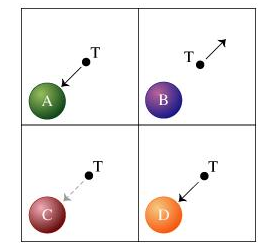# Problem: Consider three plastic balls (A, B, and C), each carrying a uniformly distributed charge equal to either +Q, -Q or zero, and an uncharged (neutral) copper ball (D). A positive test charge (T) experiences the forces shown in the figure when brought very near to the individual balls. The test charge T is strongly attracted to A, strongly repelled from B, weakly attracted to C, and strongly attracted to D.What is the nature of the force between balls A and B?a) strongly attractiveb) strongly repulsivec) weakly attractived) neither attractive nor repulsive

###### FREE Expert Solution

The basic law of Electrostatics,

Like charges repel one another while unlike charges attract one another.

The positive test charge is strongly attracted by A, meaning that A is negatively charged.

83% (136 ratings)###### Problem DetailsConsider three plastic balls (A, B, and C), each carrying a uniformly distributed charge equal to either +Q, -Q or zero, and an uncharged (neutral) copper ball (D). A positive test charge (T) experiences the forces shown in the figure when brought very near to the individual balls. The test charge T is strongly attracted to A, strongly repelled from B, weakly attracted to C, and strongly attracted to D.

What is the nature of the force between balls A and B?

a) strongly attractive

b) strongly repulsive

c) weakly attractive

d) neither attractive nor repulsive